# NCERT Solutions for Class 12 Chemistry Chapter 2 Solutions

In this chapter, we provide NCERT Solutions for Class 12 Chemistry Chapter 2 Solutions for English medium students, Which will very helpful for every student in their exams. Students can download the latest NCERT Solutions for Class 12 Chemistry Chapter 2 Solutions pdf, free NCERT solutions for Class 12 Chemistry Chapter 2 Solutions book pdf download. Now you will get step by step solution to each question. Class 12 Chemistry is a very important subject for entrance exams like IIT JEE, CPMT, etc. NCERT Class 12 chemistry notes in Hindi

## NCERT Solutions for Class 12 Chemistry Chapter 2 Solutions

INTEXT Questions

Question 1.
Calculate the mass percentage of benzene (C6H6) and carbon tetrachloride (CCI4) if 22 g of benzene is dissolved in 122 g of carbon tetrachloride.
Solution:
Mass of solution = Mass of benzene + Mass of carbon tetrachloride = 22 g +122 g = 144 g
Mass percentage of benzene =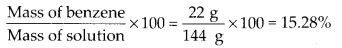∴ Mass percentage of CCl4
= 100 – Mass percentage of benzene = 100 – 15.28 = 84.72%

Question 2.
Calculate the mole fraction of benzene in solution containing 30% by mass in carbon tetrachloride.
Solution:
Let mass of solution = 100 g
∴ Mass of benzene in the solution = 30 g
∴ Mass of carbon tetrachloride = 100 – 30g = 70g
Molar mass of benzene (C6H6) = 78 g mol-1
Molar mass of CCl4 = 12 + 4 × 35.5 = 154 g mol-1
∴ No. of moles of benzeneQuestion 3.
Calculate the molarity of each of the following solutions :
(a) 30 g of Co(NO3)2.6H2O in 4.3 L of solution
(b) 30 mL of 0.5 M H2SO4 diluted to 500 mL (atomic mass of cobalt = 58.7)

Solution: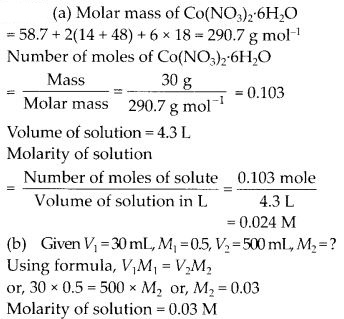Question 4.
Calculate the mass of urea (NH2CONH2) required in making 2.5 kg of 0.25 molal aqueous solution.
Solution:
Moles of urea = 0.25 mole
Mass of solvent (water) = 1 kg = 1000 g
Molar mass of urea (NH2CONH2) = 14 + 2 + 12 + 16 + 14 + 2 = 60 g mol-1
∴ Mass of urea in 1000 g of water = 0.25 mol × 60 g mol-1 = 15 g
Total mass of solution = 1000 + 15 g = 1015 g = 1.015 kg
Thus, 1.015 kg of solution contain urea = 15 g
∴ 2.5 kg of solution will require urea$\frac { 15g }{ 1.015kg }$ × 2.5 K.g = 37 g

Question 5.
Calculate (a) molality (b) molarity and (c) mole fraction of Kl if the density of 20% (mass/mass) aqueous Kl is 1.202 g mL-1

Solution: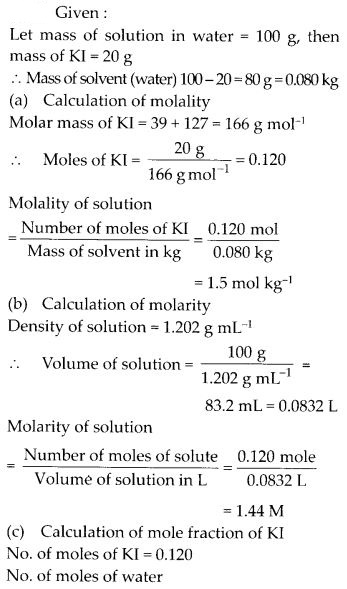Question 6.
H2S a toxic gas with rotten egg like smell, is used for qualitative analysis. If the solubility of H2S in water at STP is 0.195 m, calculate Henry’s law constant.

Solution: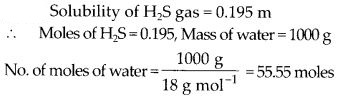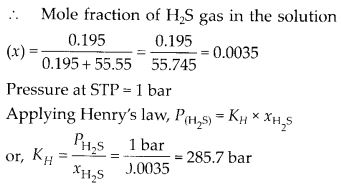Question 7.
Henry’s law constant for CO2 in water is 1.67 × 108 Pa at 298 K. Calculate the quantity of CO2 in 500 mL of soda water when packed under 2.5 atm CO2 pressure at 298K.

Solution: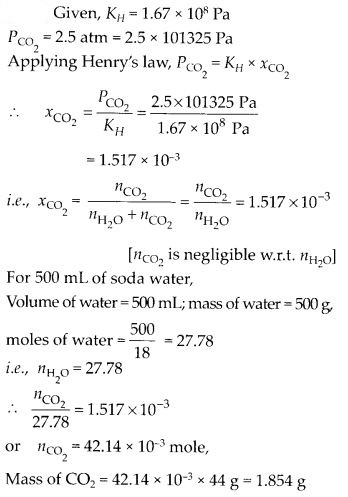Question 8.
The vapour pressures of pure liquids A and B are 450 and 700 mm Hg at 350 K respectively. Find out the composition of the liquid mixture if total vapour pressure is 600 mm Hg. Also find the composition of the vapour phase.

Solution: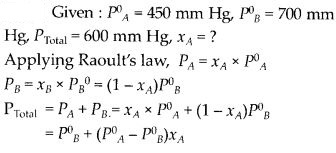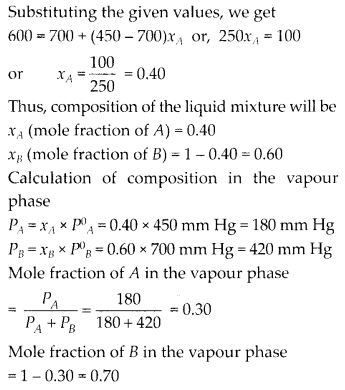Question 9.
Vapour pressure of pure water at 298 K is 23.8 mm Hg. 50 g of urea (NH2CONH2) is dissolved in 850 g of water. Calculate the vapour pressure of water for this solution and its relative lowering.

Solution:Question 10.
Boiling point of water at 750 mm Hg is 99.63°C. How much sucrose is to be added to 500 g of water such that it boils at 100°C ? Molal elevation constant for water is 0.52 K kg mol-1.

Solution: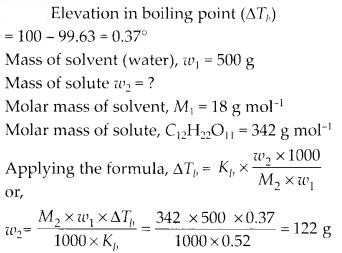Question 11.
Calculate the mass of ascorbic acid (vitamin C, C6H8O6) to be dissolved in 75 g acetic acid to lower its melting point by 1.5°C, KF = 3.9 K kg mol-1.

Solution: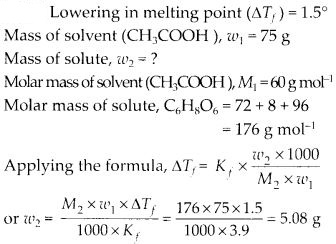Question 12.
Calculate the osmotic pressure in pascals exerted by a solution prepared by dissolving 1.0 g of polymer of molar mass 185,000 in 450 mL of water at 37°C.

Solution:NCERT Exercises

Question 1.
Define the term solution. How many types of solutions are formed? Write briefly about each type with an example.
Solution:
A solution is a homogeneous mixture of two or more pure substances which are chemically non-reacting, whose composition can be varied within certain limits. The component which is present in a large proportion in the solution is called solvent and the component present in relatively small proportion is called solute.

Types of solutions : Depending upon the physical state of solvent, solutions can be divided into three main groups :

(a) Gaseous solutions :

1. Solvent – gas, solute – gas. c.g., air (oxygen and nitrogen).
2. Solvent – gas, solute – liquid, c.g., humidity in air.
3. Solvent – gas, solute – solid, c.g., camphor in nitrogen.

(b) Liquid solutions:

1. Solvent – liquid, solute – gas. c.g., aerated drinks.
2. Solvent – liquid, solute – liquid, c.g., ethanol in water.
3. Solvent – liquid, solute – solid, c.g., salt solution in water.

(c) Solid solutions:

1. Solvent – solid, solute – gas. c.g., H2 in palladium.
2. Solvent – solid, solute – liquid, c.g., amalgam of Hg with Na.
3. Solvent – solid, solute – solid, c.g., alloys.

Question 2.
Give an example of a solid solution in which the solute is a gas.
Solution:
Solution of hydrogen in palladium and dissolved gases in minerals.

Question 3.
Define the following terms :

1. Mole fraction
2. Molality
3. Molarity
4. Mass percentage.

Solution:
(i) Mole fraction : Mole fraction of a constituent (solute as well as solvent) is the ratio of the number of moles of one component to the total number of moles of all the components present in the solution. If nA and nB are the number of moles of solvent and solute respectively then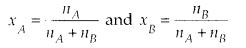(ii) Molality : Molality of a solution is defined as the number of moles of the solute dissolved in one kilogram of the solvent. It is denoted by m. It is expressed as(iii) Molarity : Molarity of a solution is defined as the number of moles of the solute dissolved in one litre or one dm3 of the solution. It is denoted by M and is expressed as(iv) Mass percentage : It may be defined as mass of solute in grams per 100 g of solution.$\frac { w }{ W }$ or mass Percentage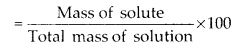Question 4.
Concentrated nitric acid used in the laboratory is 68% nitric acid by mass in aqueous solution. What should be the molarity of such a sample of the acid if the density of the solution is 1.504 g mL -1?

Solution:Question 5.
A solution of glucose in water is labelled as 10% w/w. What would be the molality and mole fraction of each component in the solution? If the density of the solution is 1.2 g mL-1, then what shall be the molarity of the solution?

Solution:Question 6.
How many mL of a 0.1 M HCI are required to react completely with 1 g mixture of Na2CO3 and NaHCO3 containing equimolar amounts of both?

Solution: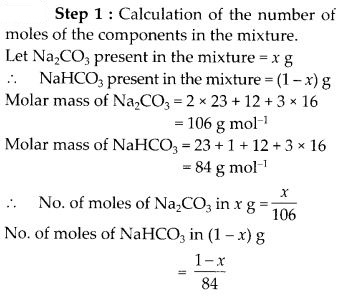Question 7.
A solution is obtained by mixing 300 g of 25% and 400 g of 40% solution by mass. Calculate the percentage of the resulting solution.
Solution:
300 g of 25% solution contains solute = 75 g
400 g of 40% solution contains solute = 160 g
Total solute = 160 + 75 = 235 g
Total solution = 300 + 400 = 700 g
% of solute in the final solution =$\frac { 235 }{ 700 }$ × 100 = 33.5%
% of water in the final solution = 100 – 33.5 = 66.5 %

Question 8.
An antifreeze solution is prepared from 222.6 g of ethylene glycol, C2H4(OH)2 and 200 g of water. Calculate the molality of the solution. If the density of the solution is 1.072 g mL-1, then what shall be the molarity of the solution?

Solution: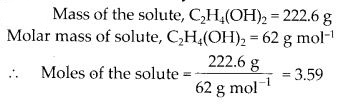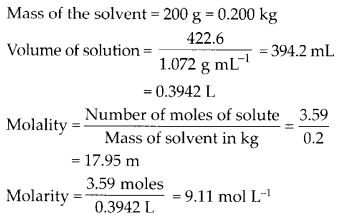Question 9.
A sample of drinking water was found to be severely contaminated with chloroform, CHCl3, supposed to be a carcinogen. The level of contamination was 15 ppm (by mass) :

1. Express this in percent by mass.
2. Determine the molality of chloroform in the water sample.

Solution: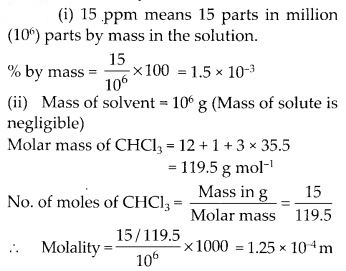Question 10.
What role does the molecular interaction play in a solution of alcohol and water ?
Solution:
There is a strong hydrogen bonding in water as well as in alcohol molecules. When alcohol and water are mixed the intermolecular hydrogen bonding between the alcohol and water molecules in the mixture is weak. Due to weaker interactions, the solution shows positive deviations from ideal behaviour. As a result, the solution will have higher vapour pressure and lower boiling point than alcohol and water separately.

Question 11.
Why do gases always tend to be less soluble in liquids as the temperature is raised ?
Solution:
As the temperature is increased, the equilibrium in a gas in liquid solution shifts in backward direction since dissolution of gas in liquid is an exothermic process. Hence the solubility of the gases decreases.

Question 12.
State Henry’s law and mention some important applications.
Solution:
Henry’s law : The solubility of a gas in a liquid at a particular temperature is directly proportional to the pressure of the gas in equilibrium with the liquid at that temperature.
or
The partial pressure of a gas in vapour phase is proportional to the mole fraction of the gas (x) in the solution. p = KHX
where KH is Henry’s law constant.
Applications of Henry’s law :
(i) To increase the solubility of CO2 in soft drinks, soda, etc., the bottles are sealed under high pressure.

(ii) In deep sea diving, increased pressure increases the solubility of atmospheric gases in blood. Oxygen is used up in the metabolism but N2remains dissolved and forms bubbles in the blood, when diver comes to the surface. To reduce this danger, N2 is replaced by He which is much less soluble in biological fluids.

(iii) In the functions of lungs – when air enters the lungs, partial pressure of oxygen is high. This oxygen combines with haemoglobin to form oxyhaemoglobin. Partial pressure of O2 in tissues is low, hence O2 is released from oxyhaemoglobin which is used for the functions of the cells.

Question 13.
The partial pressure of ethane over a saturated solution containing 6.56 × 10-3 g of ethane is 1 bar. If the solution contains 5.00 × 10-2 g of ethane, then what shall be the partial pressure of the gas?

Solution: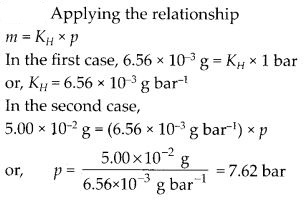Question 14.
What is meant by positive and negative deviations from Raoult’s law and how is the sign of ∆mix H related to positive and negative deviations from Raoult’s law ?

Solution: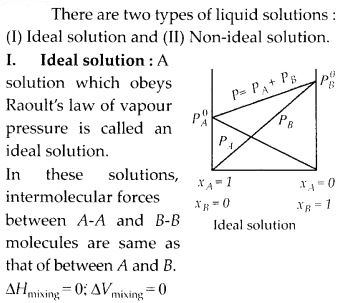Examples :

1. Benzene + toluene
2. n – Hexane + n – heptane
3. Chlorobenzene + bromobenzene
4.  Ethyl bromide + ethyl iodide

II. Non-ideal solution : A solution which does not obey Raoult’s law of vapour pressure is called non-ideal solution. They are of two types.

(a) Non-ideal solution with negative deviation : When intermolecular forces between A and B are stronger than between A-A and B-B, then intermolecular force increases, volume decreases, vapour pressure decreases and heat is released.Examples:

1. Chloroform + acetone
2. Water + sulphuric acid
3. HCl + water
4. Acetone + aniline

(b) Non-ideal solution with positive deviation: When intermolecular forces between A and B are weaker than that of A-A and B-B.

In this case intermo lecular force decreases, volume increases, vapour pressure increases, enthalpy increases.
∆V = +ve, ∆H = + ve, ∆P = + ve
So, it is called non-ideal solution with positive deviation.
PA > xA P°A, PB > xB P°B and PS > xA P°A + xB P°B
Examples :

1. Ethanol + water
2. Chloroform + ethanol

Question 15.
An aqueous solution of 2% non-volatile solute exerts a pressure of 1.004 bar at the normal boiling point of the solvent. What is the molecular mass of the solute ?
Solution:
Vapour pressure of pure water at the boiling point (P°) = 1 atm = 1.013 bar
Vapour pressure of solution (Ps) = 1.004 bar
Let mass of solution = 100 g, then, mass of solute = (W2) = 2 g
Mass of solvent (W1) = 100 – 2 = 98 g
Applying Raoult’s law for dilute solution,Question 16.
Heptane and octane form ideal solution. At 373 K, the vapour pressure of the two liquid components are 105.2 kPa and 46.8 kPa respectively. What will be the vapour pressure of a mixture of 26.0 g of heptane and 35.0 g of octane?

Solution: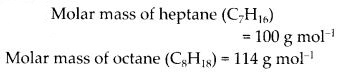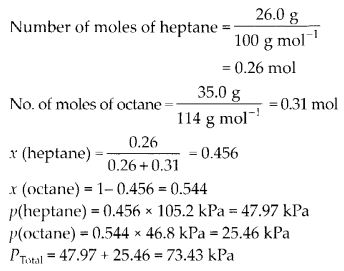Question 17.
The vapour pressure of water is 12.3 kPa at 300 K. Calculate the vapour pressure of 1 molal solution of a solute in it.

Solution:Question 18.
Calculate the mass of a non-volatile solute (molar mass 40 g mol-1) which should be dissolved in 114 g octane to reduce its vapour pressure to 80%.

Solution: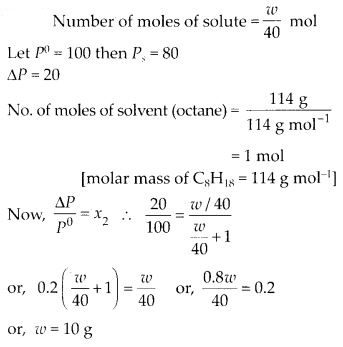Question 19.
A solution containing 30 g of non-volatile solute exactly in 90 g water has a vapour pressure of 2.8 kPa at 298 K. Further 18 g of water is then added to the solution, the new vapour pressure becomes 2.9 kPa at 298 K. Calculate

1. molar mass of the solute
2. vapour pressure of water at 298 K.

Solution: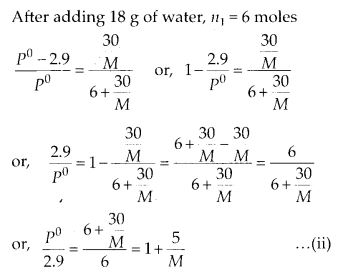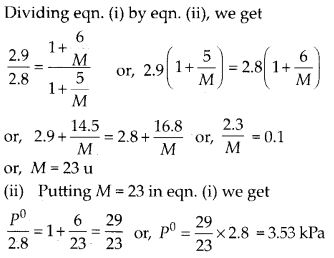Question 20.
A 5% solution (by mass) of cane sugar in water has freezing point of 271 K. Calculate the freezing point of a 5% glucose in water if freezing point of pure water is 273.15 K.

Solution: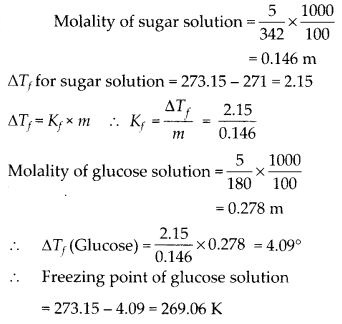Question 21.
Two elements A and 8 form compounds having molecular formula AB2 and AB4. When dissolved in 20.0 g of benzene (C6H6), 1.0 g AB2 lowers the freezing point by 2.3°C whereas 1.0 g of AB4 lowers the freezing point by 1.3 °C. The molal depression constant for benzene is 5.1 K kg mol-1. Calculate atomic mass of A and B.

Solution: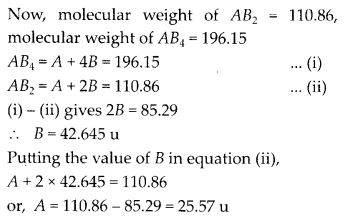Question 22.
At 300 K, 36 g of glucose present in a litre of its solution has an osmotic pressure of 4.98 bar. If the osmotic pressure of the solution is 1.52 bar at the same temperature, what would be its concentration?
Solution:
π = CRT
∴ In the first case, 4.98 =$\frac { 36 }{ 180 }$ × R × 300 = 60R ….(i)
In the second case, 1.52 = C × R × 300 …. (ii)
Dividing (ii) by (i) we get, C = 0.061 M

Question 23.
Suggest the most important type of intermolecular attractive interaction in the following pairs.

1. n – Hexane and n-octane
2. l2 and CCl4
3. NaClO4 and water
4. Methanol and acetone
5. Acetonitrile (CH3CN) and acetone (C3H6O).

Solution:

1. n-Hexane and n-octane : London dispersion forces as both the molecules are non-polar.
2. I2 and CCl4 : London dispersion forces as both the molecules are non-polar.
3. NaClO4 and water: Ion-dipole interactions as NaClO4 in water gives Na+ and ClOions. These ions interact with polar water molecules.
4. Methanol and acetone : Dipole-dipole interactions as both the molecules are polar.
5. Acetonitrile and acetone : Dipole-dipole interactions as both the molecules are polar.

Question 24.
Based on solute-solvent interactions, arrange the following in order of increasing solubility in n-octane and explain.
Cyclohexane, KCI, CH3OH, CH3CN.
Solution:

1. Cyclohexane and M-octane both are non-polar. Hence, they mix completely in all proportions.
2. KCl is an ionic compound while n-octane is non-polar. Hence, KCl will not dissolve at all in n-octane.
3. CH3OH and CH3CN both are polar but CH3CN is less polar than CH3OH. As the solvent is non-polar, CH3CN will dissolve more than CH3OH in n-octane.

Thus, the order of solubility will be KCl < CH3OH < CH3CN < Cyclohexane.

Question 25.
Amongst the following compounds, identify which are insoluble, partially soluble and highly soluble in water ?

1. Phenol,
2. Toluene,
3. Formic acid,
4. Ethyleneglycol,
5. Chloroform,
6. Pentanol.

Solution:

1. Partially soluble because phenol has polar -OH group and non-polar C6H5 group.
2. Insoluble because toluene is non-polar while water is polar.
3. Highly soluble because formic acid can form hydrogen bonds with water.
4. Highly soluble because ethylene glycol can form hydrogen bonds with water.
5. Insoluble because chloroform is an organic liquid.
6. Partially soluble because -OH group is polar but the large hydrocarbon part (C5H11) is non-polar.

Question 26.
If the density of some lake water is 1.25 g mL-1 and contains 92 g of Na+ ions per kg of water, calculate the molality of Na+ ions in the lake.

Solution: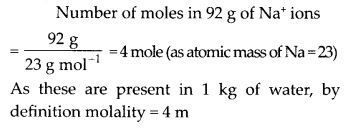Question 27.
If the solubility product of CuS is 6 × 10-16, calculate the maximum molarity of CuS in aqueous solution.

Solution: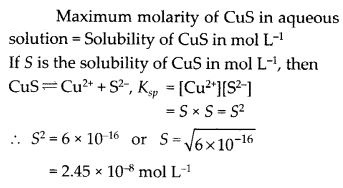Question 28.
Calculate the mass percentage of aspirin (C9H8O4) in acetonitrile (CH3CN) when 6.5 of C9H8O4 is dissolved in 450 g of CH3CN.

Solution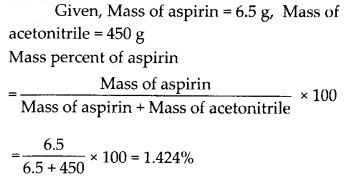Question 29.
Nalorphene (C19H21NO3), similar to morphine, is used to combat withdrawal symptoms in narcotic users. Dose of nalorphene generally given is 1.5 mg. Calculate the mass of 1.5 × 10-3 m aqueous solution required for the above dose.

Solution: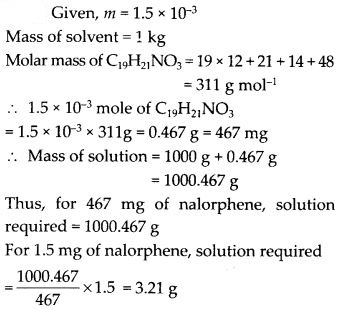Question 30.
Calculate the amount of benzoic acid (C6H5COOH) required for preparing 250 mL of 0.15 M solution in methanol.

Solution:Question 31.
The depression in freezing point of water observed for the same amount of acetic acid, trichloroacetic acid and trifluoroacetic acid increases in the order as given above. Explain briefly.
Solution:
The depression in freezing point is in the order :
Acetic acid (CH3 – COOH) < trichloroacetic acid (Cl3C – COOH)
< trifluoroacetic acid (F3C – COOH)
Fluorine, being most electronegative, has the highest electron withdrawing inductive effect. Consequently, trifluoroacetic acid is the strongest acid while acetic acid is the weakest acid. Hence, trifluoroacetic acid ionizes to the largest extent while acetic acid ionizes to the minimum extent to give ions in their solutions in water. Greater the ions produced, greater is the depression in freezing point. Hence, the depression in freezing point is the maximum for the fluoroacetic acid and minimum for acetic acid.

Question 32.
Calculate the depression in the freezing point of water when 10 g of CH3CH2CHCICOOH is added to 250 g of water.
(Ka = 1.4 × 10-3, Kf = 1.86 K kg mol-1)

Solution:Question 33.
19.5 g of CH2FCOOH is dissolved in 500 g of water. The depression in the freezing point observed is 1.0°C. Calculate the van’t Hoff factor and dissociation constant of fluoroacetic acid. Kf for water is 1.86 K kg mol-1.

Solution: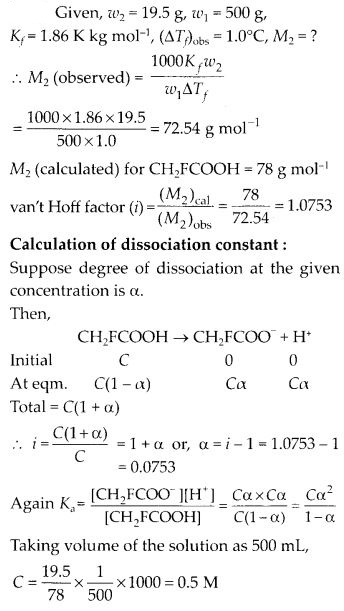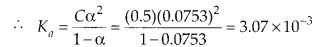Question 34.
Vapour pressure of water at 293 K is 17535 mm Hg. Calculate the vapour pressure of water at 293 K when 25 g of glucose is dissolved in 450 g water.

Solution: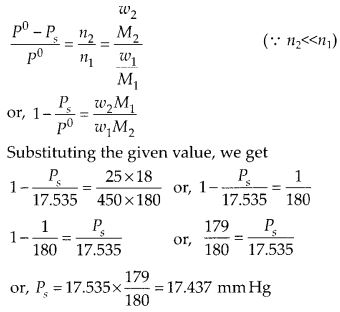Question 35.
Henry’s law constant for the molality of methane in benzene at 298 K is 4.27 × 105 mm Hg. Calculate the solubility of methane in benzene at 298 K under 760 mm Hg.

Solution: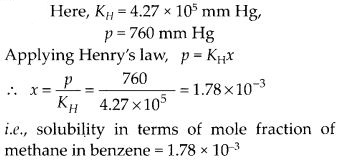Question 36.
100 g of liquid A (molar mass 140 g mol-1) was dissolved in 1000 g of liquid B (molar mass 180 g mol-1). The vapour pressure of pure liquid B was found to be 500 torr. Calculate the vapour pressure of pure liquid A and its vapour pressure in the solution if the total vapour pressure of the solution is 475 torr.

Solution: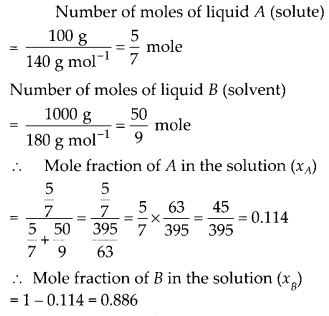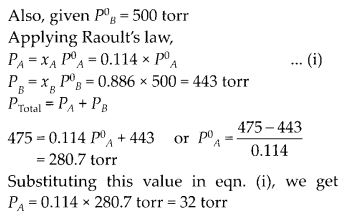Question 37.
Vapour pressure of pure acetone and chloroform at 328 K are 741.8 mm Hg and 632.8 mm Hg respectively. Assuming that they form ideal solution over the entire range of composition, plot Ptotal, Pchloroform and Pacetone as a function of Xacetone. The experimental data observed for different compositions of mixtures is :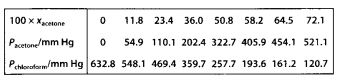Plot this data also on the same graph paper. Indicate whether it has positive deviation or negative deviation from the ideal solution.
Solution: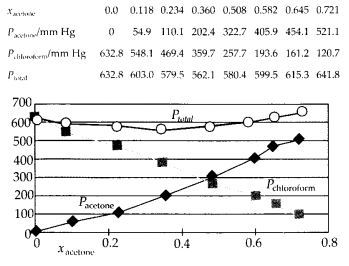As the plot for Ptotal dips downwards, hence the solution shows negative deviation from the ideal behaviour.

Question 38.
Benzene and naphthalene form ideal solution over the entire range of composition.The vapour pressures of pure benzene and naphthalene at 300,K are 50.71 mm Hg and 32.06 mm Hg respectively. Calculate the mole fraction of benzene in the vapour phase if 80 g of benzene is mixed with 100 g of naphthalene.

Solution: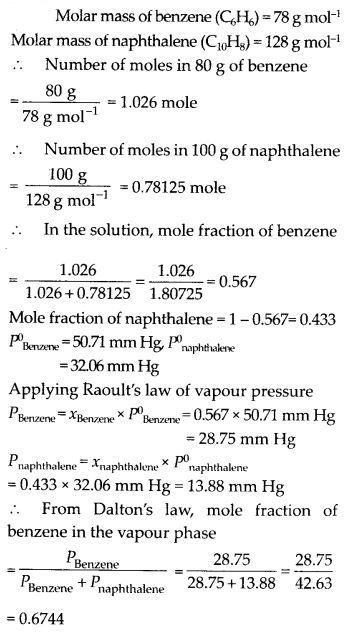Question 39.
The air is a mixture of a number of gases. The major components are oxygen and nitrogen with approximate proportion of 20% and 79% by volume at 298 K. The water is in equilibrium with air at a pressure of 10 atm. At 298 K, if the Henry’s law constants for oxygen and nitrogen are 3.30 × 107 mm Hg and 6.51 × 107 mm Hg respectively, calculate the composition of these gases in water.

Solution:Question 40.
Determine the amount of CaCl2 (i = 2.47) dissolved in 2.5 litre of water such that its osmotic pressure is 0.75 atm at 27°C.

Solution: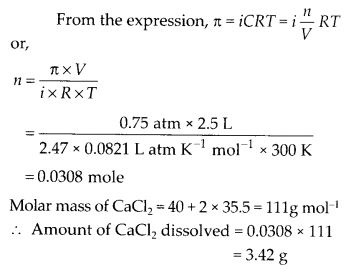Question 41.
Determine the osmotic pressure of a solution prepared by dissolving 25 mg of K2SO4 in 2 litre of water at 25°C, assuming that it is completely dissociated.

Solution: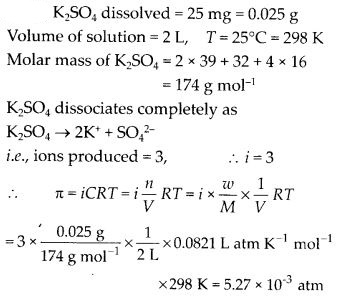All Chapter NCERT Solutions For Class12 Chemistry

—————————————————————————–

All Subject NCERT Solutions For Class12

*************************************************

Remark:

I think you got complete solutions for this chapter. If You have any queries regarding this chapter, please comment on the below section our subject teacher will answer you. We tried our best to give complete solutions so you got good marks in your exam.

If these solutions have helped you, you can also share Careerkundali.in to your friends.

Best of Luck!!